﻿ 高铁车站行人过闸行为仿真及参数校核 Pedestrian Behavior Simulation Crossing Automatic Ticket Check Machine and Parameters Calibration

Open Journal of Transportation Technologies
Vol. 09  No. 02 ( 2020 ), Article ID: 34738 , 9 pages
10.12677/OJTT.2020.92012

Pedestrian Behavior Simulation Crossing Automatic Ticket Check Machine and Parameters Calibration

Jinrui Liu*, Jinshu Li

School of Traffic and Transportation Engineering, Central South University, Changsha Hunan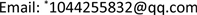Received: Mar. 3rd, 2020; accepted: Mar. 19th, 2020; published: Mar. 26th, 2020ABSTRACT

In the face of peak travel demand during the Spring Festival and other rush periods, how to ensure that passengers check in on train station within the specified time is a problem worth studying. As the bottleneck zone when the pedestrian flow inside the station hub passes, the efficiency of pedestrian ticket checking directly affects the operational efficiency of the high-speed rail station, and which is also an important factor in determining the station scale. This article studies the pedestrian crossing behavior from micro-simulation perspective. First, the social force model of the passengers carrying small luggage passengers, medium and large luggage passengers and passengers of different ages was established. Then, through the statistical analysis of the behavior of the passengers in the Changsha High-speed Railway South Station, the important parameters of the model are calibrated before the behavior of the pedestrian ticket checking is simulated. Finally, the actual and simulated gate time data are compared and analyzed to verify the effectiveness of the passengers’ social force model.

Keywords:Social Force Model, Pedestrian Crossing Auto Gate, Parameter Correction, Pedestrian Simulation, Hypothesis Testing1. 引言

2. 传统社会力模型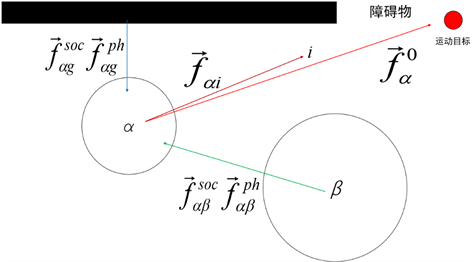Figure 1. Traditional pedestrian stress diagram

${m}_{\alpha }\frac{\text{d}{w}_{\alpha }\left(t\right)}{\text{d}t}={F}_{\alpha }\left(t\right)+\epsilon$ (1)

${F}_{\alpha }\left(t\right)={F}_{\alpha }^{0}\left({v}_{\alpha },{v}_{\alpha }^{0}{e}_{\alpha }\right)+{\sum }_{\beta }{F}_{\alpha \beta }\left({e}_{\alpha },{r}_{\alpha }-{r}_{\beta }\right)+{\sum }_{g}{F}_{\alpha g}\left({e}_{\alpha },{r}_{\alpha }-{r}_{\beta }\right)$ (2)

${F}_{\alpha }^{0}\left(t\right)={m}_{\alpha }\frac{{v}_{\alpha }^{0}\left(t\right){e}_{\alpha }^{0}\left(t\right)-{v}_{\alpha }\left(t\right)}{{\tau }_{\alpha }}$ (3)

${F}_{\alpha \beta }={f}_{\alpha \beta }^{soc}\left(t\right)+{f}_{\alpha \beta }^{ph}\left(t\right)$ (4)

${F}_{\alpha g}={f}_{\alpha g}^{soc}\left(t\right)+{f}_{\alpha g}^{ph}\left(t\right)$(5)

3. 乘客自主过闸社会力模型

${F}_{\alpha }\left(t\right)={F}_{\alpha }^{0}+{\sum }_{\beta }{F}_{\alpha \beta }+{F}_{\alpha G}+\epsilon$ (6)

${F}_{\alpha G}={F}_{\alpha F}+{F}_{\alpha g}+{F}_{pg}$ (7)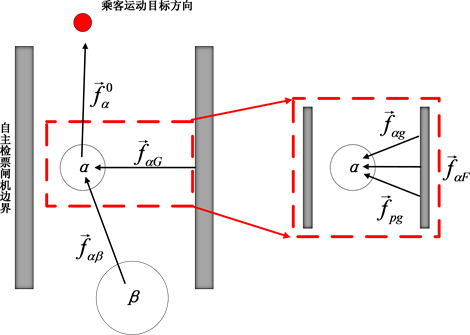Figure 2. Schematic diagram of the force when the passenger passes the gate

3.1. 小件行李乘客过闸社会力模型

${F}_{\alpha small}\left(t\right)={F}_{\alpha small}^{0}\left(t\right)+{\sum }_{\beta }{F}_{\alpha small\beta }\left(t\right)+{F}_{\alpha smallg}\left(t\right)+\epsilon$ (8)

${F}_{\alpha small}\left(t\right)={m}_{\alpha }\frac{{v}_{\alpha }^{0}\left(t\right){e}_{\alpha }^{0}\left(t\right)-{v}_{\alpha }\left(t\right)}{{\tau }_{\alpha }}+{\sum }_{\beta }\left({f}_{\alpha \beta }^{soc}\left(t\right)+{f}_{\alpha \beta }^{ph}\left(t\right)\right)+{f}_{\alpha g}\left(t\right)+\epsilon$ (9)

3.2. 中大件行李乘客过闸社会力模型

${F}_{\alpha big}\left(t\right)={F}_{\alpha big}^{0}\left(t\right)+{\sum }_{\beta }{F}_{\alpha big\beta }\left(t\right)+{F}_{\alpha \alpha bigg}\left(t\right)+{F}_{Pg}\left(t\right)+\epsilon$ (10)

${F}_{Pg}\left(t\right)=kg\left({r}_{Pg}-{d}_{Pg}\right){n}_{Pg}+ug\left({r}_{Pg}-{d}_{Pg}\right)\Delta {v}_{P\alpha }^{t}{t}_{PG}$ (11)

3.3. 老年乘客过闸社会力模型

${F}_{\alpha old}\left(t\right)={F}_{\alpha old}^{0}+{\sum }_{\beta }{F}_{\alpha old\beta }+{F}_{\alpha oldg}+\epsilon$(12)

4. 实验数据及参数校核

4.1. 样本概况与预处理Table 1. Pedestrian crossing behavior survey sample summary table

4.2. 乘客过闸时间分析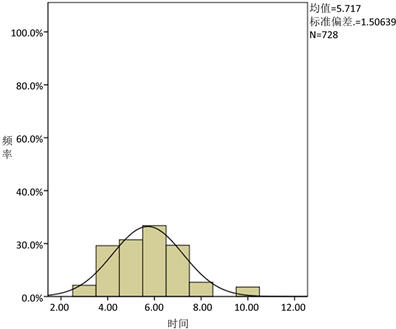Figure 3. Passenger crossing time distribution mapTable 2. Distribution of different types of passengers

4.3. 参数校正中关键指标获取Table 3. Different types of passenger speed expectation zone

4.4. 最优参数确定

5. 乘客过闸社会力模型仿真

1) 乘客类型

2) 乘客数量输入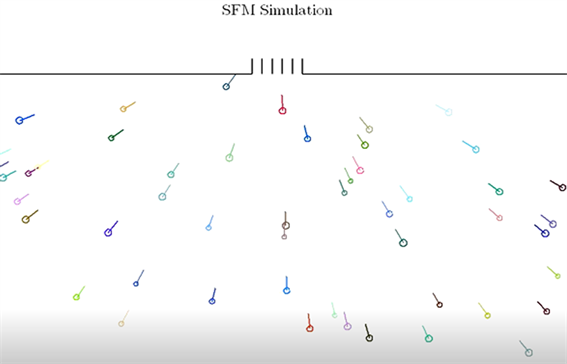Figure 4. Initial state of passengers before crossing

6. 模型检验

${a}_{\alpha }=\frac{{F}_{\alpha }\left(t\right)}{{m}_{\alpha }}$ (13)

${t}_{\alpha }=\sqrt{\frac{2s}{{a}_{\alpha }}}$ (14)

${a}_{\alpha }$$\alpha$ 乘客过闸的加速度； ${F}_{\alpha }\left(t\right)$$\alpha$ 乘客过闸时的社会力； ${m}_{\alpha }$$\alpha$ 乘客的质量； ${t}_{\alpha }$$\alpha$ 乘客的过闸时间；s为闸机通道长度。Table 5. Small baggage passengers crossing time sample statistical descriptionTable 6. Analysis of differences in passing time of small baggage passengers

7. 结论

1) 对乘客过闸行为特性及影响因素进行研究分析发现：男、女乘客过闸时间不存在显著差异，而不同年龄之间存在显著差异；是否携带行李是影响乘客过闸时的重要因素之一，携带小件行李乘客过闸时间与携带中大件行李乘客的过闸时间之间具有显著的差异；影响过闸效率的第三个因素为过闸流程熟练程度。引导老人及携带中大件行李乘客标线从人工通道通行是提高匝机通行效率的措施。

2) 针对影响乘客过闸的三个主要因素：行李、年龄、对过闸流程熟练程度造成延误三个因素，建立了乘客过闸社会力模型。利用matlab编程仿真，以及SPSS数据分析仿真所得过闸时间与现实调研统计过闸时间之间的差异性，检验了乘客过闸社会力模型的科学、有效性。

Pedestrian Behavior Simulation Crossing Automatic Ticket Check Machine and Parameters Calibration[J]. 交通技术, 2020, 09(02): 101-109. https://doi.org/10.12677/OJTT.2020.92012

1. 1. Joseph, S., Milazzo, I.I., Nagui, M., Rouphail, et al. (1999) Quality of Service for Uninterrupted Pedestrian Facilities in the 2000 Highway Capacity Manual. The 78th Annual Meeting of the Transportation Research Board, North Carolina State, 1 January 1999, 18-24. https://doi.org/10.3141/1678-03

2. 2. Oxley, J.A., et al. (2005) Crossing Road Safely: An Expe-rimental Study of Age Differences in Gap Selection by Pedestrians. Accident Analysis & Prevention, 37, 962-971. https://doi.org/10.1016/j.aap.2005.04.017

3. 3. 陈然, 董力耘. 中国大都市行人交通特征的实测和初步分析[J]. 上海大学学报(自然科学版), 2005, 11(1): 93-97.

4. 4. Helbing, D. and Mulnar, P. (1995) Social Force Model for Pedestrian Dynamics. Physical Review E, 51, 1282-1286. https://doi.org/10.1103/PhysRevE.51.4282

5. 5. 刘明姝, 张国宝. 基于排队系统的城市轨道交通进站检票机配置[J]. 城市轨道交通研究, 2004, 7(5): 34-37.

6. 6. 覃松涛, 叶霞飞. 衔接铁路客运站的城市轨道交通车站闸机通过能力分析[J]. 城市轨道交通研究, 2013, 1(5): 100-103.

7. 7. 翟向荣. 城市轨道交通车站设备通行能力匹配性研究[D]: [硕士学位论文]. 北京: 北京交通大学, 2012.

8. 8. Helbing, D., Farkas, I. and Vicsek, T. (2000) Simulating Dynamical Features of Escape Panic. Nature, 407, 487-490. https://doi.org/10.1038/35035023

9. 9. Muramatsu, M. and Nagatani, T. (2000) Jamming Transition of Pedestrian Traf-fic at a Crossing with Open Boundaries. Physica A: Statistical Mechanics and Its Applications, 286, 377-390. https://doi.org/10.1016/S0378-4371(00)00336-8

10. 10. 熊英男. 城市轨道交通车站自动检票闸机通行能力分析与配置数量研究[D]: [硕士学位论文]. 成都: 西南交通大学, 2016.

11. 11. 李永行. 城市轨道交通车站行人微观行为建模与仿真[D]: [硕士学位论文]. 长春: 吉林大学, 2018.

12. 12. 龙泓玥. 城市轨道交通进站自动检票机配置优化研究[D]: [硕士学位论文]. 成都: 西南交通大学, 2017.